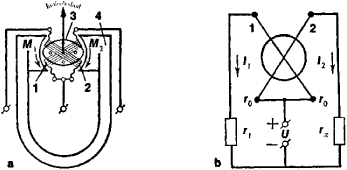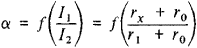# Quotient Meter

The following article is from The Great Soviet Encyclopedia (1979). It might be outdated or ideologically biased.

## Quotient Meter

a device in instruments used to measure the ratio of two currents. The principle of operation of a quotient meter is based on the balancing of opposing torque moments—arising from the action on the moving part of the values whose ratio is being measured—upon deflection of the moving part through a certain angle. For example, the moving part of a permanent-magnet quotient meter is composed of two frames, mounted at an angle, to which current is fed by zero-torque coils (Figure 1,a). Since the frames are located in the field of a permanent magnet, they tend to turn in the direction of greater torque moment. The moving part of the quotient meter will deflect until the moments are in equilibrium.Figure 1. (a) Diagram of a permanent-magnet quotient meter; (b) schematic diagram of an ohmmeter with a permanent-magnet quotient meter: (M1) and (M2) torque moments, (I1) and (I2) currents in the circuits of the ohmmeter, (U) power supply, (r0) resistance of the frames of the quotient meter, (r1) ohmic resistance, (rx) resistance being measured, (1) and (2) frames of the quotient meter, (3) core, (4) permanent magnet

Quotient meters are widely used in various circuits for measuring electrical quantities, such as capacitance, inductance, or resistance. For example, if a quotient meter is used in an ohmmeter (Figure l,b), the angle of deflection α of the moving part depends only on the ratio of the currents I1 and I2.That Is, for constant r0 and r1 the deflection of the moving part is proportional to the resistance being measured. The scale of a quotient meter may be calibrated directly in ohms. Quotient meters with electrodynamic and ferrodynamic systems are also widely used.